Function Repository Resource:

# ComplexToPolar

Get a complex number in polar form or polar exponential form

Contributed by: Wolfram|Alpha Math Team
 ResourceFunction["ComplexToPolar"][z] returns the complex number z in polar exponential form. ResourceFunction["ComplexToPolar"][z,type] returns the complex number z with the form type.

## Details and Options

For numeric input, ResourceFunction["ComplexToPolar"] returns a complex number in an Inactive form. For symbolic input, ResourceFunction["ComplexToPolar"] returns unevaluated.
Possible types include All, "Polar" and "Exponential".
The polar form of a complex number is z=r(cosθ+i sinθ) where r and θ are real.
The exponential form of a complex number is z=reiθ where r and θ are real.

## Examples

### Basic Examples (4)

Return a polar exponential form for a numeric quantity:

 In:=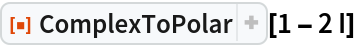Out=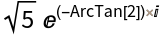Specify that you want the number in polar form:

 In:=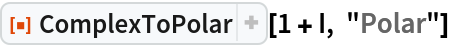Out=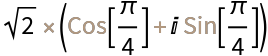Specify that you want the number in polar exponential form:

 In:=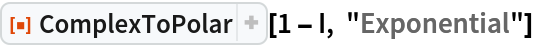Out=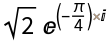Return all forms as an Association:

 In:=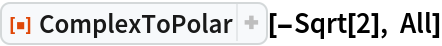Out=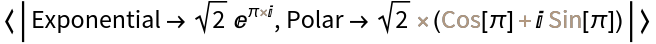### Scope (1)

For symbolic input, ComplexToPolar returns unevaluated:

 In:=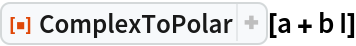Out=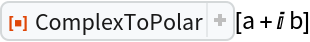## Publisher

Wolfram|Alpha Math Team

## Version History

• 2.0.0 – 23 March 2023
• 1.0.0 – 11 May 2020

## Author Notes

To view underlying source code, evaluate the following:

 In:=